# Dropout技术介绍2019年4月26日07:40:25

3 3732字阅读12分26秒

## Dropout在做什么

### Train时Dropout在做什么

dropout(p)时，意味着每个neuron, 有p%的可能性被去除;（这里需要注意的是，不是去除p%的neuron）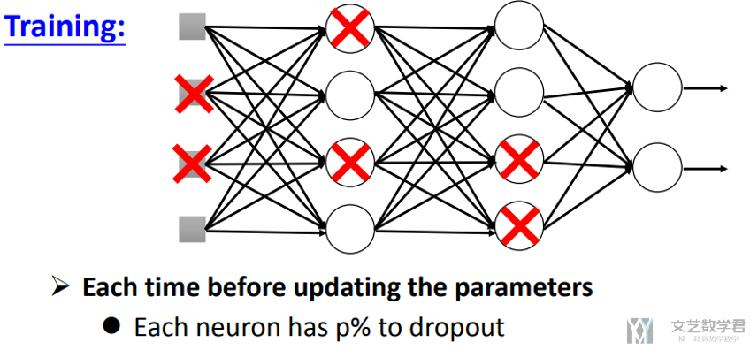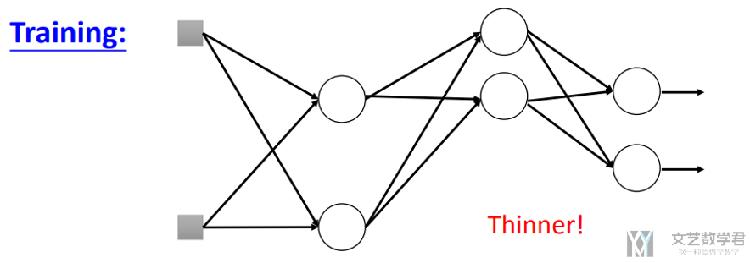• 每次前向传播时, 去除一些neuron;
• 网络的结构发生了改变;
• 使用新的网络结构进行训练;
• 对于每一个min-batch, 每次都会修改网络的结构;

### Test时Dropout在做什么

• 不进行dropout（即每个节点都不会被去掉）;
• 同时, 若train时dropout(p), 则在test时候, 所有系数需要乘(1-p%);
• 例如, dropout(0.9), 则最后所有系数需要乘(1-0.9)；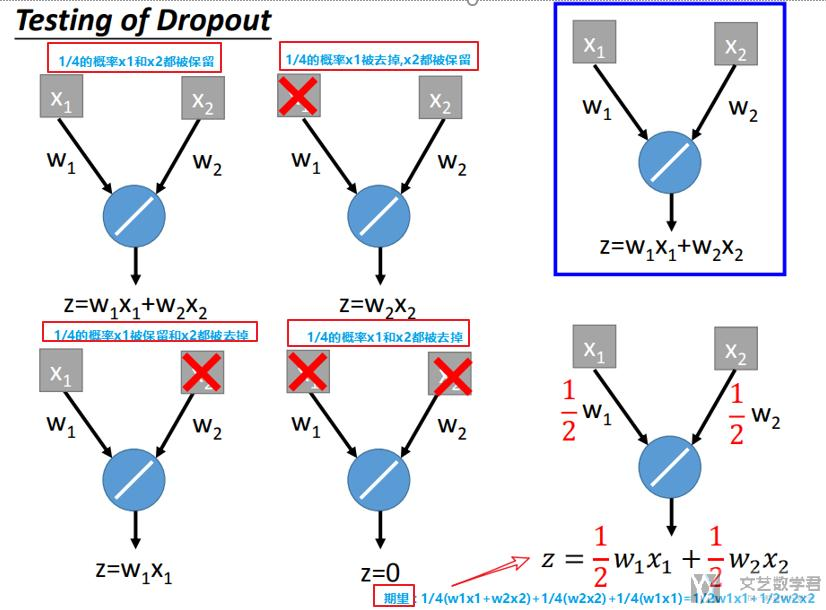### Pytorch手动实现Dropout

• z = 2×w1×x1+2×w2×x2
• z = 2×w1×x1
• z = 2×w2×x2
• z = 0

1. def dropout_layer(X, dropout):
2.     assert 0 <= dropout <= 1
3.     # In this case, all elements are dropped out
4.     if dropout == 1:
5.         return torch.zeros_like(X)
6.     # In this case, all elements are kept
7.     if dropout == 0:
8.         return X
9.     mask = (torch.Tensor(X.shape).uniform_(0, 1) > dropout).float()
10.     return mask * X / (1.0-dropout)

1. X= torch.arange(16, dtype = torch.float32).reshape((2, 8))
2. print(X)
3. """
4. tensor([[ 0.,  1.,  2.,  3.,  4.,  5.,  6.,  7.],
5.         [ 8.,  9., 10., 11., 12., 13., 14., 15.]])
6. """
7. print(dropout_layer(X, 0.5))
8. """
9. tensor([[ 0.,  0.,  0.,  0.,  8., 10.,  0., 14.],
10.         [16., 18.,  0., 22.,  0., 26.,  0., 30.]])
11. """

1. class Net(nn.Module):
2.     def __init__(self, num_inputs, num_outputs, num_hiddens1, num_hiddens2, is_training = True):
3.         super(Net, self).__init__()
4.         self.num_inputs = num_inputs
5.         self.is_training = is_training
6.         self.lin1 = nn.Linear(num_inputs, num_hiddens1)
7.         self.lin2 = nn.Linear(num_hiddens1, num_hiddens2)
8.         self.lin3 = nn.Linear(num_hiddens2, num_outputs)
9.         self.relu = nn.ReLU()
10.     def forward(self, X):
11.         H1 = self.relu(self.lin1(X.reshape((-1, self.num_inputs))))
12.         # Use dropout only when training the model
13.         if self.is_training == True:
14.             # Add a dropout layer after the first fully connected layer
15.             H1 = dropout_layer(H1, dropout1)
16.         H2 = self.relu(self.lin2(H1))
17.         if self.is_training == True:
18.             # Add a dropout layer after the second fully connected layer
19.             H2 = dropout_layer(H2, dropout2)
20.         out = self.lin3(H2)
21.         return out

## Dropout有用的一种解释

Dropout相当于是一种ensemble的技术，因为每一个batch训练的网络结构是不同的，所以最终结果相当于是很多网络结构的ensemble.

### 要注意的是

• dropout需要样本量比较多；
• 过拟合的时候使用dropout技术，即training set上很好, testing set上结果不好。
• 在使用dropout的时候，可以适当增加模型的层数（复杂度）

## Dropout的具体实现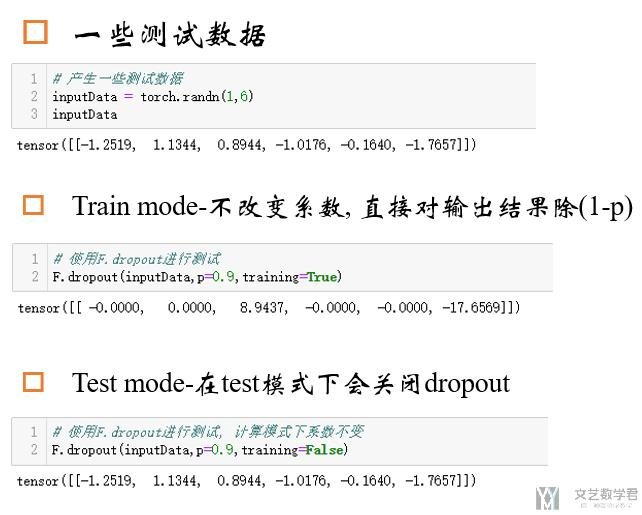1. model.train()  # Set model to training mode
2. model.eval()   # Set model to evaluate mode

## Pytorch实验

### 网络结构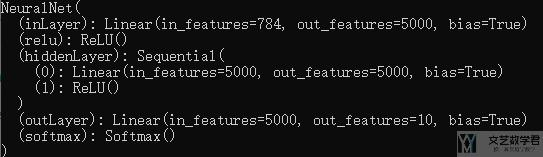### 不使用Dropout技术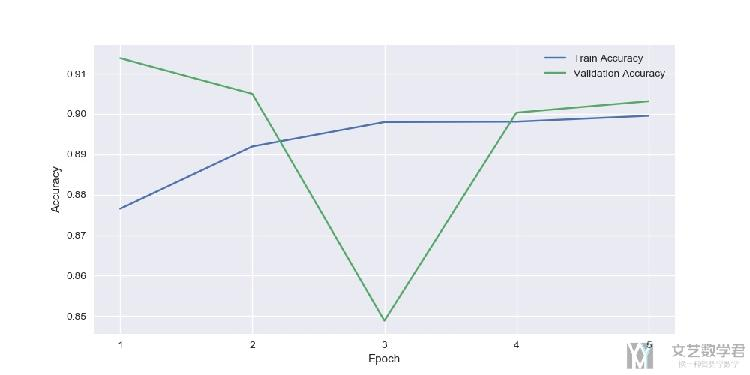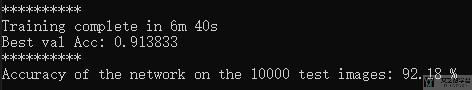### 使用Dropout技术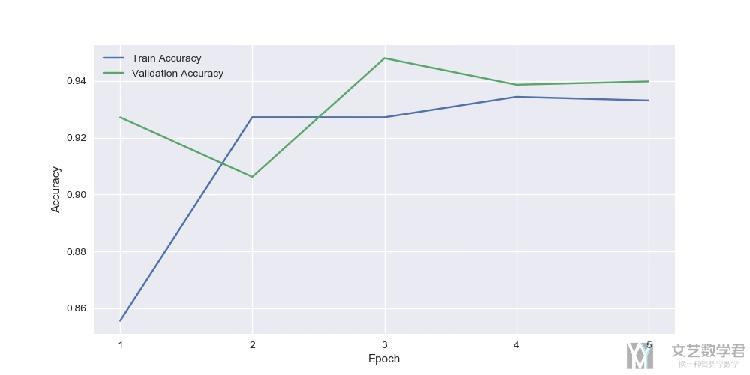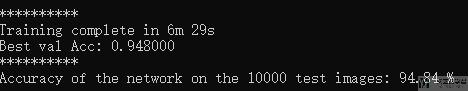## 结语• 微信公众号
• 关注微信公众号
•• QQ群
• 我们的QQ群号
•• 本文由 发表于 2019年4月26日07:40:25
• 转载请务必保留本文链接：https://mathpretty.com/10332.html